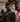# NYU Bridge to Tandon - Week 7

August 20, 2020

## Problem Solving with C++, 9.1

A pointer is the memory address of a variable. If we declare `int x = 6;` a few things happen. The number 6 is created somewhere in memory (stack), then we assign a name to identify it. That name in a human way to read the location of that value. Calling a variable is the same as telling the machine “please go to this location and tell me what is stored there”.

A pointer can be stored directly in a variable, known as a pointer variable. We declare these with the star sign, such as `int *p` - which is, p is the memory address of an integer. When we assign values to a pointer variable we do so by getting the address of another variable using the address-of operator.

``````// declare a pointer variable for an int

int &p;

// declare a variable
int x = 5;

// tell p to point  to the location of x
p = &x;``````

Pointers would be useless if all they could do is point to a location. We’d like to tell the computer “please go to this location and get me the variable there”. We call this dereferencing and it is called with the * operator again.

``````int x = 0;
int *p = &x;

*p = 42; // now we told the computer "go to the location at p and change the value to 42

cout << *p << endl; // this is now 42
cout << x << endl; // this is now 42``````

``````int *p1, *p2;
int v1 = 0;

p1 = &v1;
p2 = p1; // p2 now points to v1's address``````

We don’t actually need to even create a variable to point to in order for a pointer to be valid. We can use the `new` keyword to assign a pointer to a location holding an int. When we use the new keyword, we are creating a dynamic variable

``int *p = new *int;``

Dynamic variables need to be held somewhere. That portion of unallocated memory is called the heap or freestore. It is possible to fill the heap with too many variables, which will cause the next call to `new` to crash the program. In order to prevent this crash, we use the `delete` keyword to free memory used by a variable.

``````int *p = new int;

*p = 10;

cout << *p << endl; //prints 10

delete p; // now the value of p is nothing``````

Note that we delete the pointer, not the dereferencing. Once a pointer is deleted, it is called a dangling pointer - don’t dereference these!

The opposite of a dynamic program, one that has its lifetime handled by the compiler, is an automatic variable or ordinary variable.

We can use `typedef` to alias types to apply reader-semantics or domain-information to a type. That is `typedef double Kilometer` would allow us to then say `Kilometer distance = 123.45;`

This also works for pointers. If we wanted to say that, for example, this pointer is pointing to an int on the heap, we could say `typedef int* IntPtr;` This also lets you declare multiple variables of pointer type easily, such as `IntPtr p1, p2;`

If you want to pass a pointer into a function by reference, you need the & sign to determine reference and * to annotate pointer type.

``````void functionthing(&*int ptr);

// or
typedef *int IntPtr;

void otherfn(&IntPtr ptr);``````

## Problem Solving with C++, 9.2

A dynamic array is one that is heap-allocated and can grow and shrink in size according to the number of elements in the array.

We can think of the variable of an array as a pointer since it refers to the address of the first element in the array, but it CANNOT be reassigned to a pointer (unlike a real pointer). That is

``````int a;
typedef *int IntPtr;
IntPtr p;

p = a; // valid! P can point to a

a = p; // invalid!! a is not of type *int``````

To allocate an array on the heap, we use the `new` keyword like

``````double *p = new double ;

// do stuff with array

delete [] p; // array notation to delete an array pointer``````

Note that deleting a pointer to an array must be `delete [] ptr;` - this tells the system to delete the entirety of the length of the array, rather than the first element.

We can use pointer arithmetic to index an array, that is

``````double *p = new double ;

for (int i = 0; i <10; i++ ) {
// go to location p, adds i * sizeof(double) and get value
cout << *(p + i) << endl;
} ``````I work and live in Brooklyn, NY building software.